# Sequences and Series Notes Class 11th Maths

0
2244

1. Sequence: Sequence is a function whose domain is a subset of natural numbers. It represents the images of 1, 2, 3,… ,n, as f1, f2, f3, …., fn , where fn = f(n).

2. Real Sequence: A sequence whose range is a subset of R is called a real sequence.

3. Series: If a1, a2, a3 , … , an is a sequence, then the expression a1 + a2 + a3 + … + an is a series.

4. Progression: A sequence whose terms follow certain rule is called a progression.

5. Finite Series: A series having finite number of terms is called finite series.

6. Infinite Series: A series having infinite number of terms is called infinite series.

Arithmetic Progression (AP)

A sequence in which the difference of two consecutive terms is constant, is called Arithmetic Progression (AP).

Properties of Arithmetic Progression

(i) If a sequence is an AP, then its nth term is a linear expression in n, i.e., its nth term is given by An + B, where A and B are constants and A = common difference.

(ii) nth Term of an AP If a is the first term, d is the common difference and / is the last term of an AP, then

(a) nth term is given by 1= an = a + (n – 1)d

(b) nth term of an AP from the last term is a’n = l – (n – 1)d

(c) an + a’n = a + 1
i.e., nth term from the start + nth term from the end
= constant
= first term + last term

(d) Common difference of an AP
d = Tn – Tn-1, ∀ n > 1

(e) Tn = 1/2[Tn-k + Tn+k], k < n

(iii) If a constant is added or subtracted from each term of an AP, then the resulting sequence is an AP with same common difference.

(iv) If each term of an AP is multiplied or divided by a non-zero constant k, then the resulting sequence is also an AP, with common difference kd or d/k where d = common difference.

(v) If an, an+1 and an+2 are three consecutive terms of an AP, then 2an+1 = an + an+2.

(vi) (a) Any three terms of an AP can be taken as a – d, a, a + d.

(b) Any four terms of an AP can be taken as a-3d,a- d, a + d, a + 3d.

(c) Any five terms of an AP can be taken as a-2d,a – d, a, a + d, a + 2d.

(vii) Sum of n Terms of an AP

(a) Sum of n terms of AP, is given by Sn = n/2[2a + (n – 1)d] = n/2[a + l]

(b) A sequence is an AP, iff the sum of n terms is of the form An2 + Bn, where A and B are constants. Common difference in such case will be 2A.

(c) Tn = Sn – Sn-1

(viii) a2, b2 and c2 are in AP.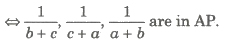(ix) If a1, a2,…, an are the non-zero terms of an AP, then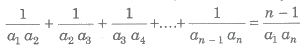(x) Arithmetic Mean

(a) If a, A and b are in AP, then A= (a + b)/2 is called the 2 arithmetic mean of a and b.

(b) If a1, a2, a3 , an are n numbers, then their AM is given by,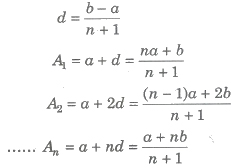(c) If a, A1 , A2 , A3 ,…,An, b are in AP, then A1, A2, A3,…, An are n arithmetic mean between a and b, where(d) Sum of n AM’s between a and b is nA
i.e., A1 + A2 + A3 + + = nA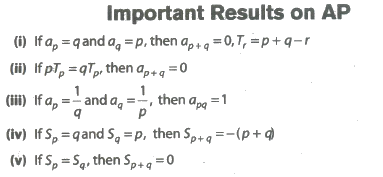Geometric Progression (GP)

A sequence in which the ratio of two consecutive terms is constant is called GP. The constant ratio is called common ratio (r).
i.e., an+1/an = r, ∀ n ≥ 1

Properties of Geometric Progression (GP)

(i) nth Term of a GP If a is the first term and r is the common ratio

(a) nth term of a GP from the beginning is an = arn-1
(b) nth term of a GP from the end is a’n = l/rn-1, l = last term
(c) If a is the first term and r is the common ratio of a GP, then the GP can be written as a, ar, ar2,… , arn-1, …
(d) The nth term from the end of a finite GP consisting of m terms is arm-n, where a is the first term and r is the common ratio of the GP.
(e) ana’n = al i.e., nth term from the beginning x nth term from the end = constant = first term x last term.

(ii) If all the terms of GP be multiplied or divided by same non-zero constant, then the resulting sequence is a GP with the same common ratio.

(iii) The reciprocal terms of a given GP form a GP.

(iv) If each term of a GP be raised to same power, the resulting sequence also forms a GP.

(v) If the terms of a GP are chosen at regular intervals, then the resulting sequence is also a GP.

(vi) If a1, a2, a3, … , an are non-zero, non-negative term of a GP, then

(a) GM = (a1a2a3… an )1/n
(b) log a1, log a2, log a3,…, log an are in an AP and vice-versa.

(vii) If a, b and c are three consecutive terms of a GP, then b2 = ac

(viii) (a) Three terms of a GP can be taken as a/r, a and ar.
(b) Four terms of a GP can be taken as a/r3, a/r, ar and ar3.
(c) Five terms of a GP can be taken as a/r2, a/r, ar and ar2.

(ix) Sum of n Terms of a GP

(a) Sum of n terms of a GP is given by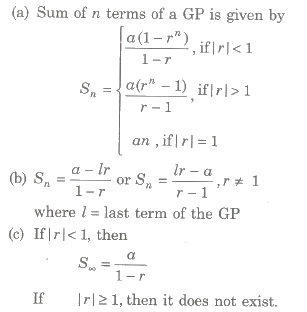(x) Geometric Mean (GM)

(a) If a, G, b are in GP, then G is called the geometric mean of a and b and is given by G = √ab
(b) If a, G1, G2, G3, , Gn, b are in GP, then G1, G2, G3,… , Gn, are in GM’s between a and b, where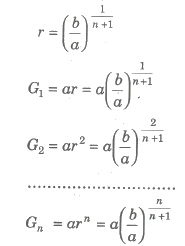(c) Product of n GM’s, G1 X G2 X G3 X … X Gn = Gn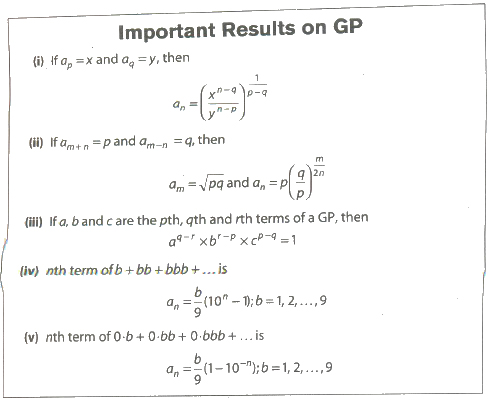Harmonic Progression (HP)

A sequence a1, a2, a3 ,…, an of non-zero numbers is called a Harmonic Progression (HP), if the sequence 1/a1, 1/a2, 1/a3, …, 1/an is an AP.

Properties of Harmonic Progression (HP)

(i) nth term of HP, if a1, a2, a3 ,…, an are in HP, then
(a) nth term of the HP from the beginning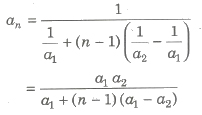(b) nth term of the HP from the end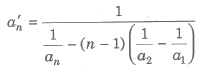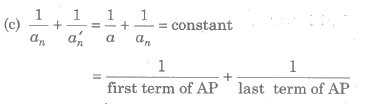(d) an = 1/a+(n-1)d are the first term and common difference of the corresponding AP.
(ii) Sum of harmonic progression does not exist.

Harmonic Mean

(i) If a, H,b are in HP, then H is called the harmonic mean of a and b i.e., H= 2ab/(a + b)

(ii) If a, H1, H2, H3, …, Hn, b are in HP, then
H1, H2, H3, …, Hn

are n harmonic means between a and b where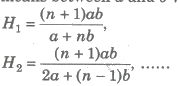(iii) Harmonic Mean (HM) between a1, a2, a3, …, an is given by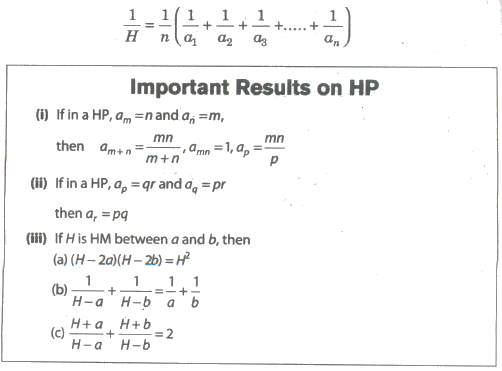Properties of AM, GM and HM between Two Numbers

If A, G and H are arithmetic, geometric and harmonic means of two positive numbers a and b, then
(i) A=(a+b)/2, G=√ab, H=(2ab)/(a+b)
(ii) A≥G≥H
(iii) A, G, H are in GP and G2 = AH
(iv) If A,G,H are AM, GM and HM between three given numbers a, b and c, then the equation on having a, b and c as its root is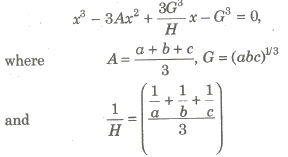(v) If A1,A2 be two AM’s, G1, G2 be two GM’s and H1, H2 be two HM’s between two numbers a and b, then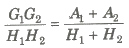(vi) If A,G and H be AM, GM and HM between two numbers a and b, then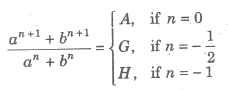Arithmetico-Geometric Progression

A sequence in which every term is a product of a term of AP and GP is known as arithmetico-geometric progression.

The series may be written as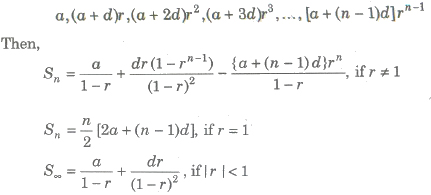Sum of Arithmetico-Geometric Series

Type 1 Let al + a2 + a3 + … be a given series. If a2 – al, a3 – a2, … are in AP or GP, then an and Sn can be found by the method of difference.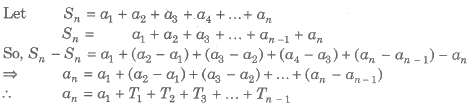where T1, T2, T3 ,… are terms of new series and Sn = Σan

Type 2 It is not always necessary that the series of first order of differences i.e., a2 – a1, a3 – a2, …, an – an-1 is always either in AP or in GP in such case.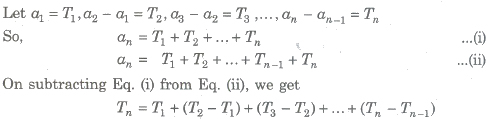Now, the series (T2 — T1) + (T3 — T2) + …+ (Tn – Tn-1) is series of second order of differences and when it is either in AP or in GP, then an = a1 + ΣTr

Otherwise, in the similar way, we find series of higher order of differences and the nth term of the series.

Exponential Series

The sum of the series is denoted by the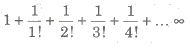number e.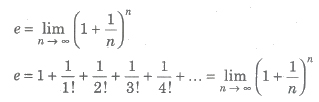(i) e lies between 2 and 3.
(ii) e is an irrational number.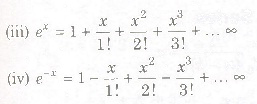Exponential Theorem

Let a>0, then for all real value of x,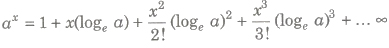Logarithmic Series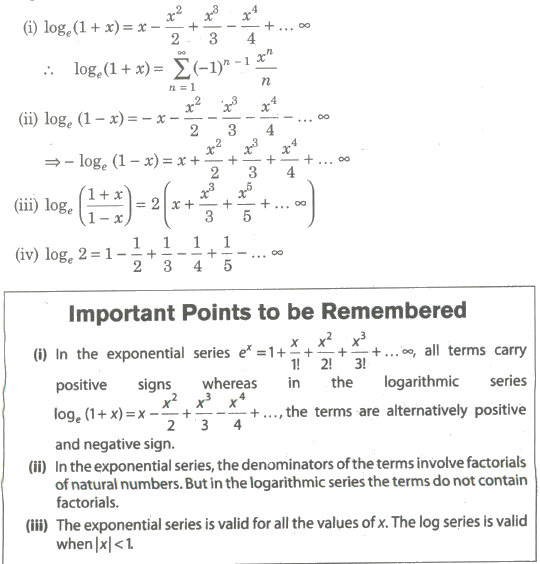Important Result and Useful Series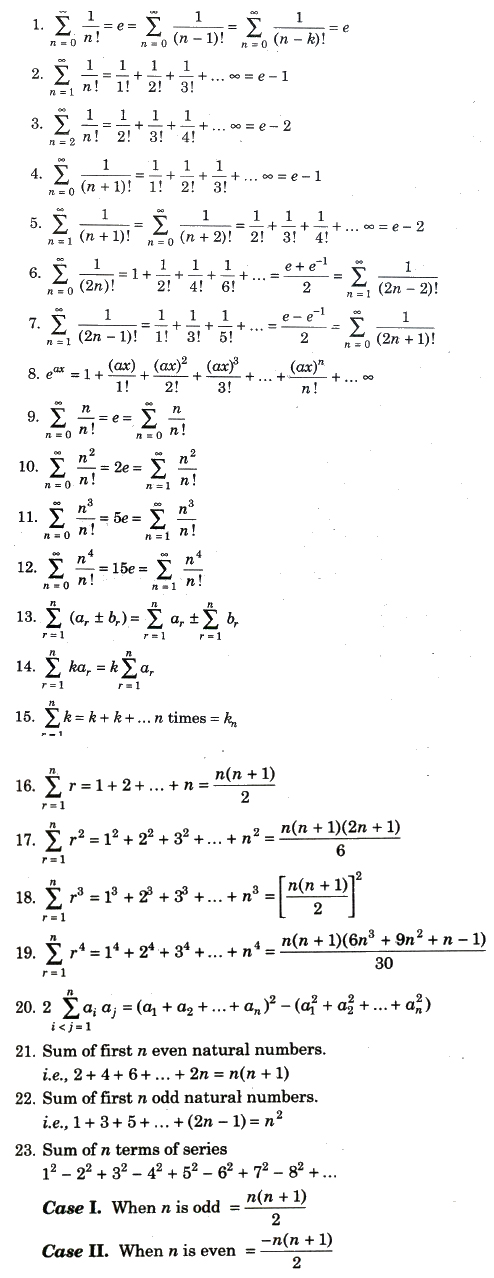24. If number of terms in AP/GP/HP are odd, then AM/GM/HM of first and last term in middle term of progression.

25. If pth, qth and rth term of geometric progression are also in geometric progression.

26. If a,b and c are in AP and also in GP, then a=b=c

27. If a, b and c are in AP, then xa, xb and xc are in geometric progression.

Previous articleQuadratic Equations and Inequalities Notes Class 11th Maths